### Individual project:

Redraw an echo hello-world board, add (at least) a button and LED (with current-limiting resistor) check the design rules, make it, and test it.

I started taking the instructions from Alex page to download first the files and then I decided to work with Eagle.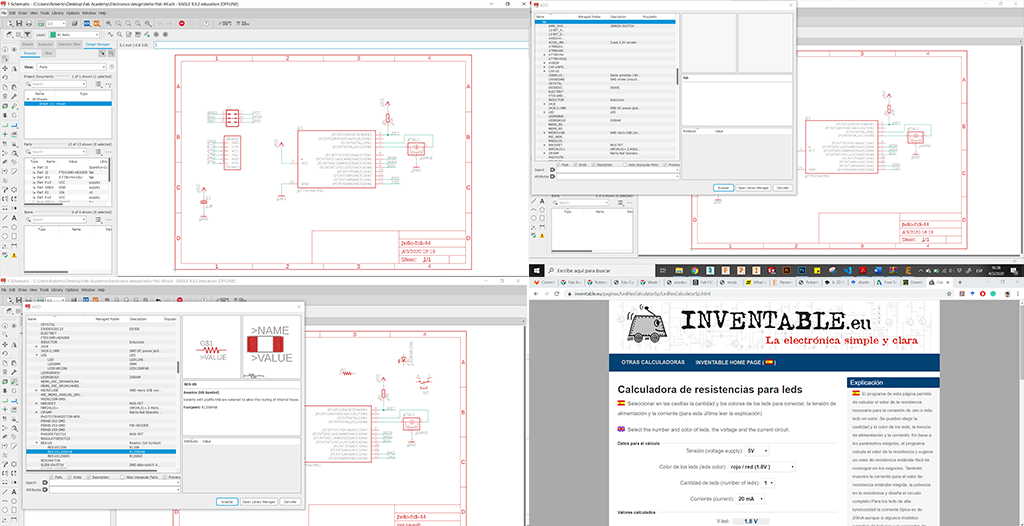Then, this is the work with libraries and connections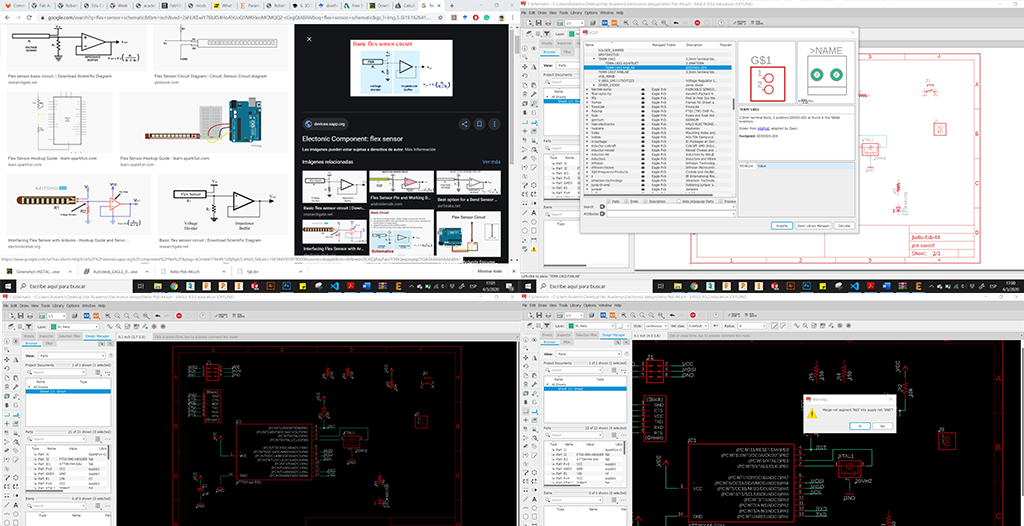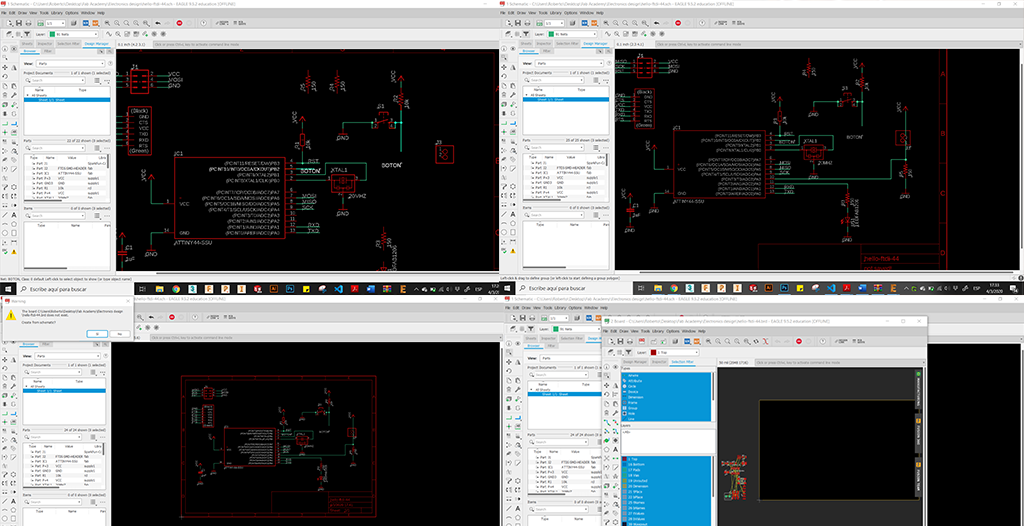I proceed with the design.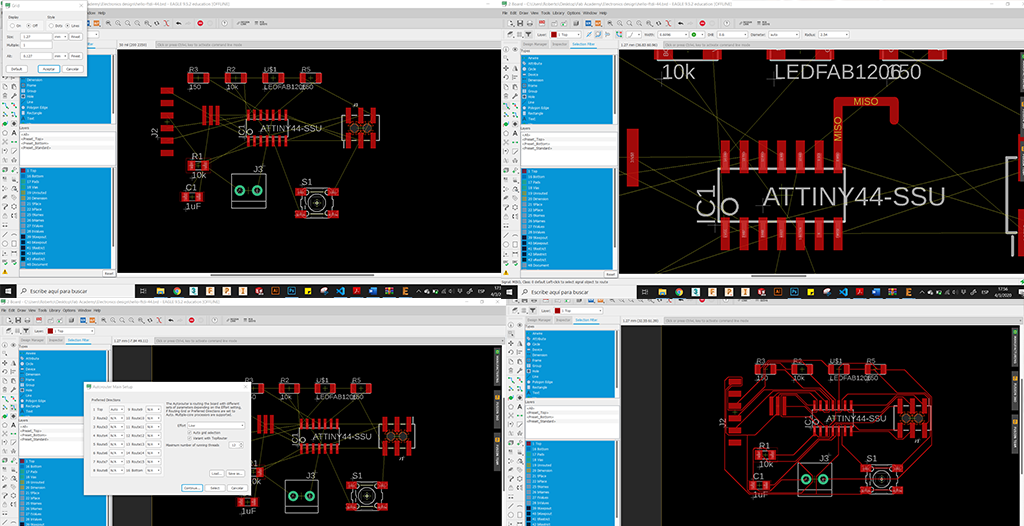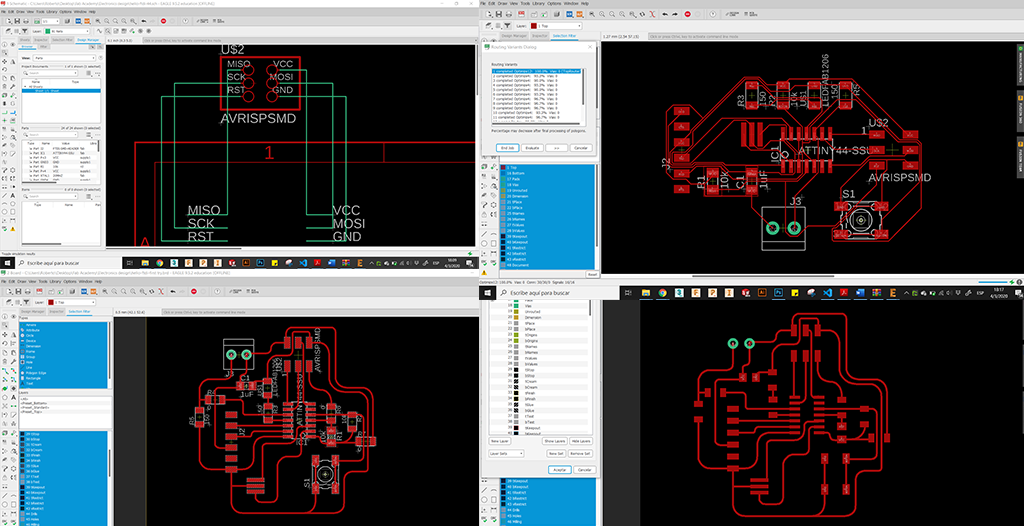Getting the G code ready with mods.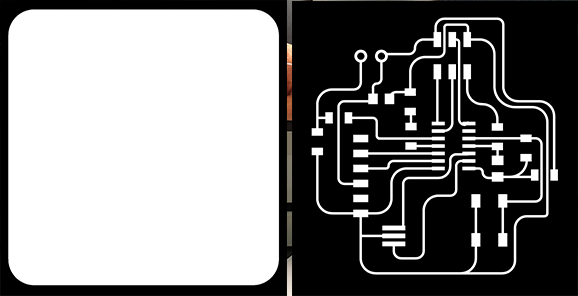Milling and soldering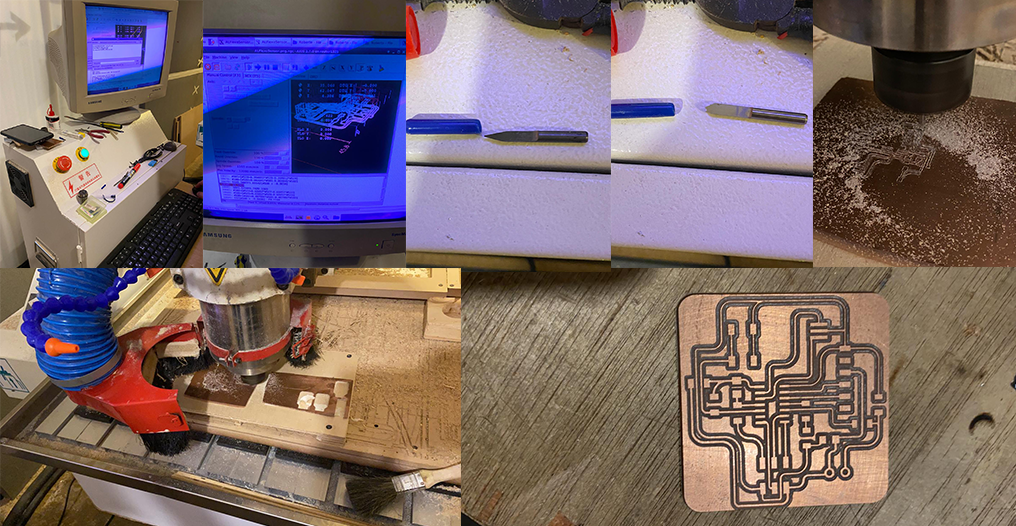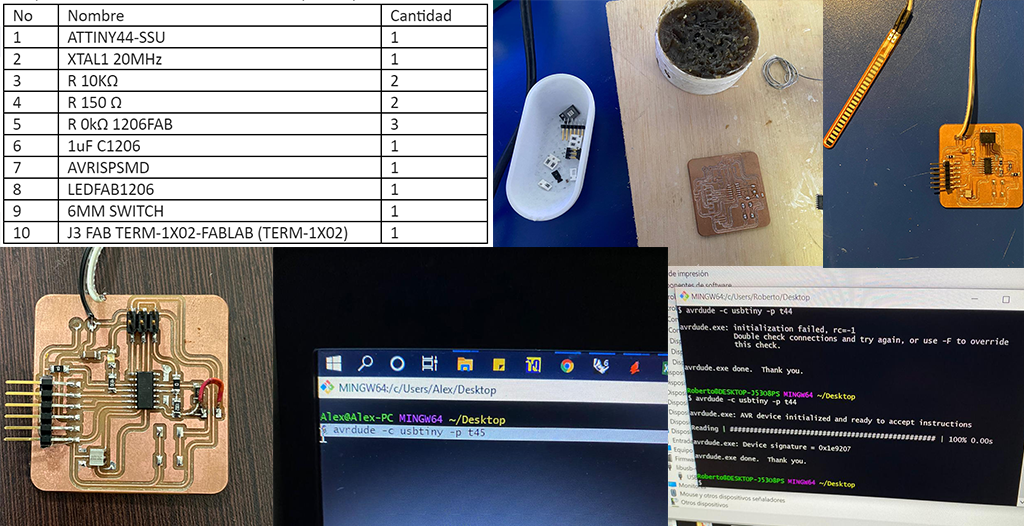On-line programming helping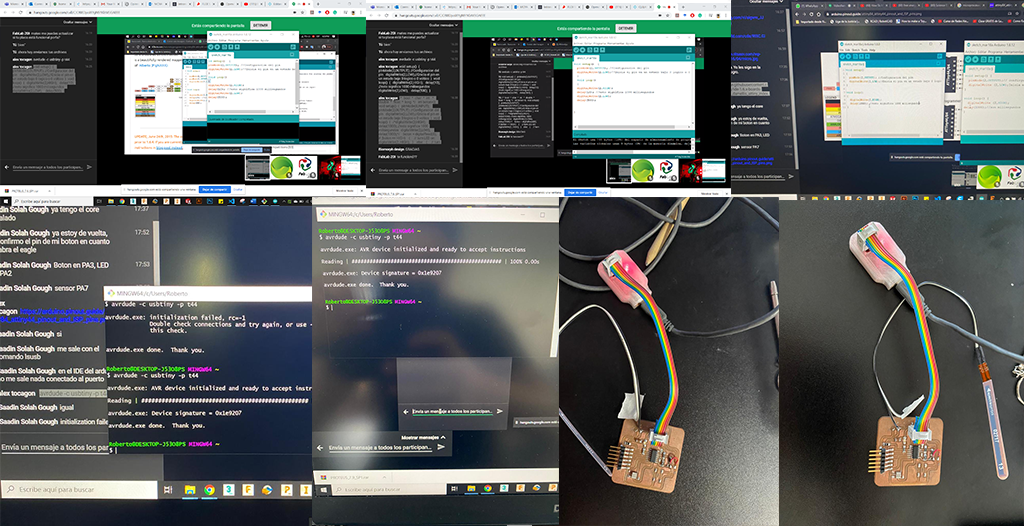Arduino, LCD, Servo TEST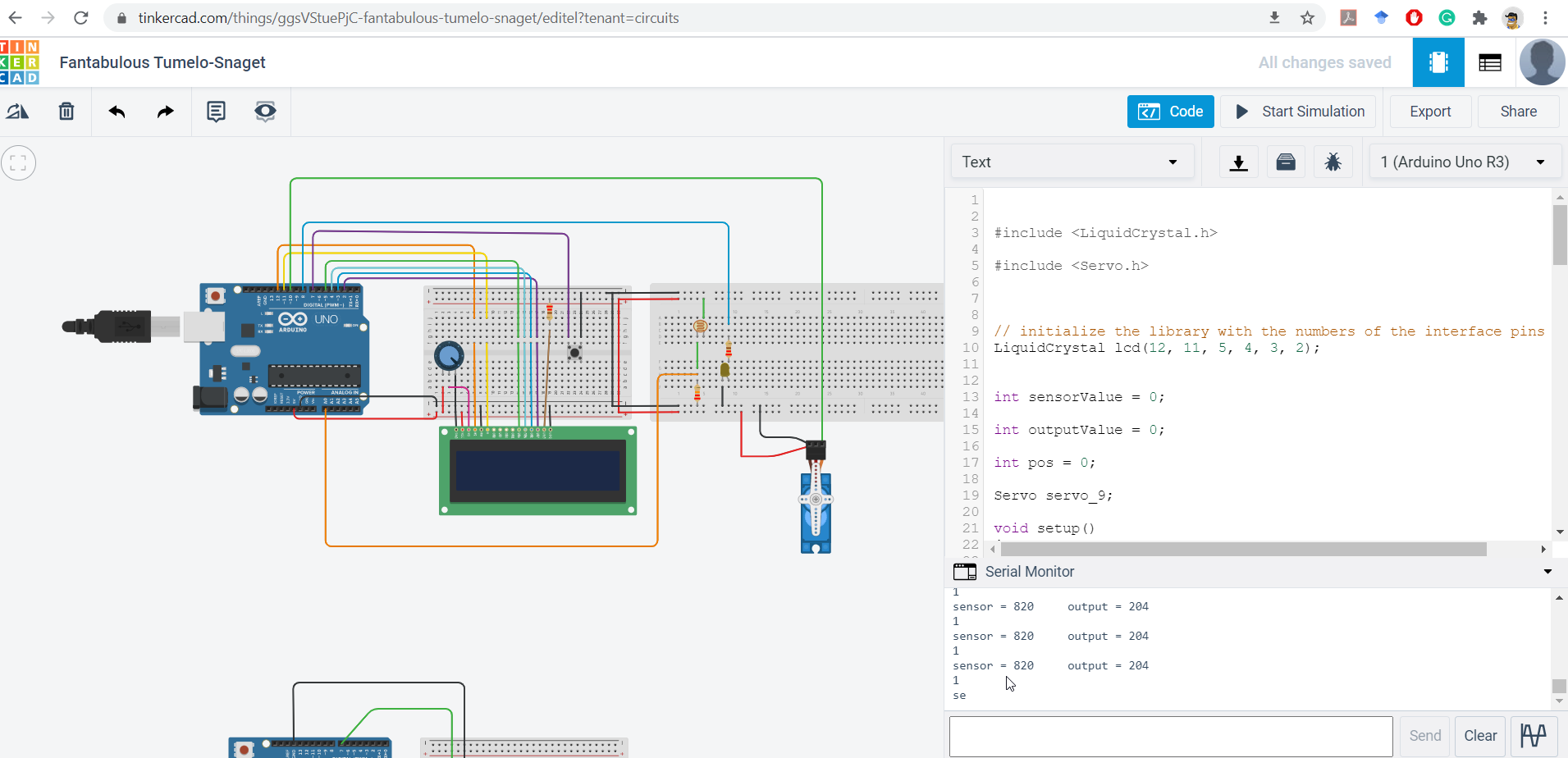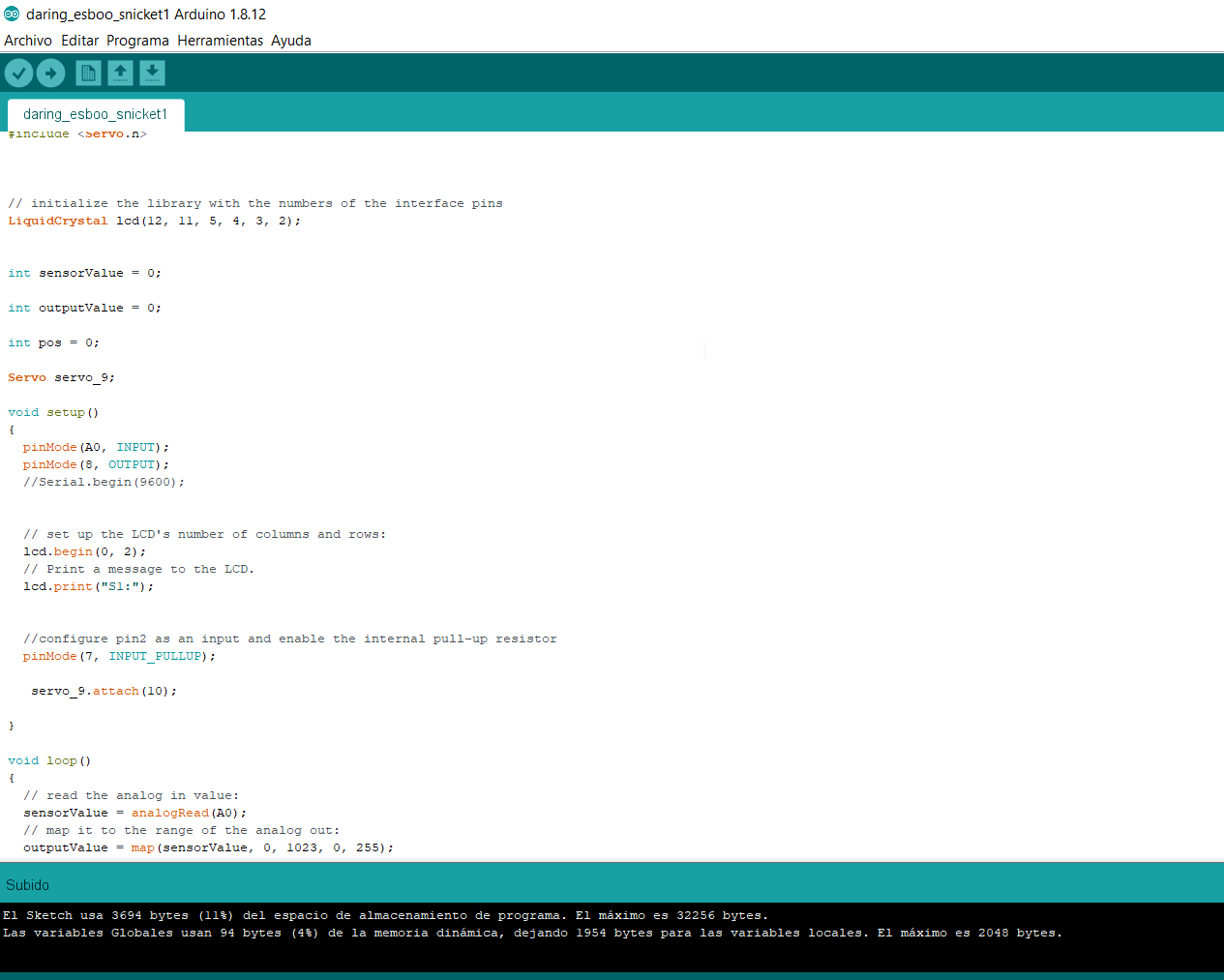``````//Firs Try
//972020
#include

#include

// initialize the library with the numbers of the interface pins
LiquidCrystal lcd(12, 11, 5, 4, 3, 2);

int sensorValue = 0;

int outputValue = 0;

int pos = 0;

Servo servo_9;

void setup()
{
pinMode(A0, INPUT);
pinMode(8, OUTPUT);
//Serial.begin(9600);

// set up the LCD's number of columns and rows:
lcd.begin(0, 2);
// Print a message to the LCD.
lcd.print("S1:");

//configure pin2 as an input and enable the internal pull-up resistor
pinMode(7, INPUT_PULLUP);

servo_9.attach(10);

}

void loop()
{
// map it to the range of the analog out:
outputValue = map(sensorValue, 0, 1023, 0, 255);
// change the analog out value:
analogWrite(8, outputValue);
// print the results to the serial monitor:
//Serial.print("sensor = ");
//Serial.print(sensorValue);
//Serial.print("\t output = ");
//Serial.println(outputValue);
// wait 2 milliseconds before the next loop for the
// analog-to-digital converter to settle after the
delay(2); // Wait for 2 millisecond(s)

// Low: 0 - 402
// Medio: 403 - 510
// High: mayor o igual 511

if (sensorValue>=0 && sensorValue <=402)
{
lcd.setCursor(0, 1);
// print the number of seconds since reset:
lcd.print("L");
}

if (sensorValue>=403 && sensorValue <=510)
{
lcd.setCursor(0, 1);
// print the number of seconds since reset:
lcd.print("M");
}

if (sensorValue>=511)
{
lcd.setCursor(0, 1);
// print the number of seconds since reset:
lcd.print("H");
}

// set the cursor to column 0, line 1
// (note: line 1 is the second row, since counting begins with 0):
////  lcd.setCursor(0, 1);
// print the number of seconds since reset:
///  lcd.print(sensorValue);

// lcd.setCursor(6, 1);
//  lcd.print(outputValue);

//read the pushbutton value into a variable
//print out the value of the pushbutton
// Serial.println(sensorVal);

// Keep in mind the pullup means the pushbutton's
// logic is inverted. It goes HIGH when it's open,
// and LOW when it's pressed. Turn on pin 13 when the
// button's pressed, and off when it's not:

servo_9.write(0);

if (sensorVal == LOW)
{
//digitalWrite(13, LOW);
servo_9.write(180);
}

}

``````

## Conclusion

• Double check PCB tracks before applying power.
• When designing the tracks on the computer it is necessary to take it easy, for me this was one of the biggest drawbacks in this process.
• It is not necessary to be an expert in the use of digital tools, it is advisable to review the information of previous students who had similar problems.
• To make the cut in the CNC milling machine the best option is to do a test before applying the cut, especially if you have never used this machine before.
• After having several problems with programming I took the option of doing the programming in the Arduino simulator and this made the process much easier.